Ex.3.7 (Optional)

Chapter 3 Class 10 Pair of Linear Equations in Two Variables
Serial order wise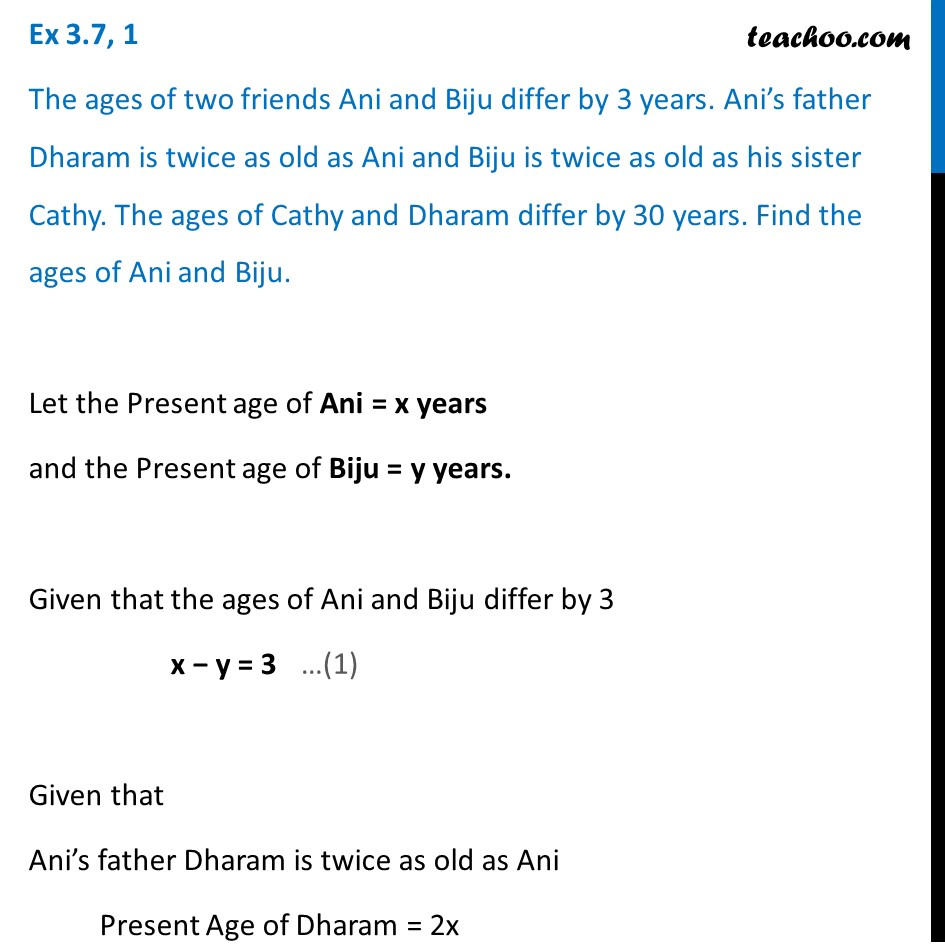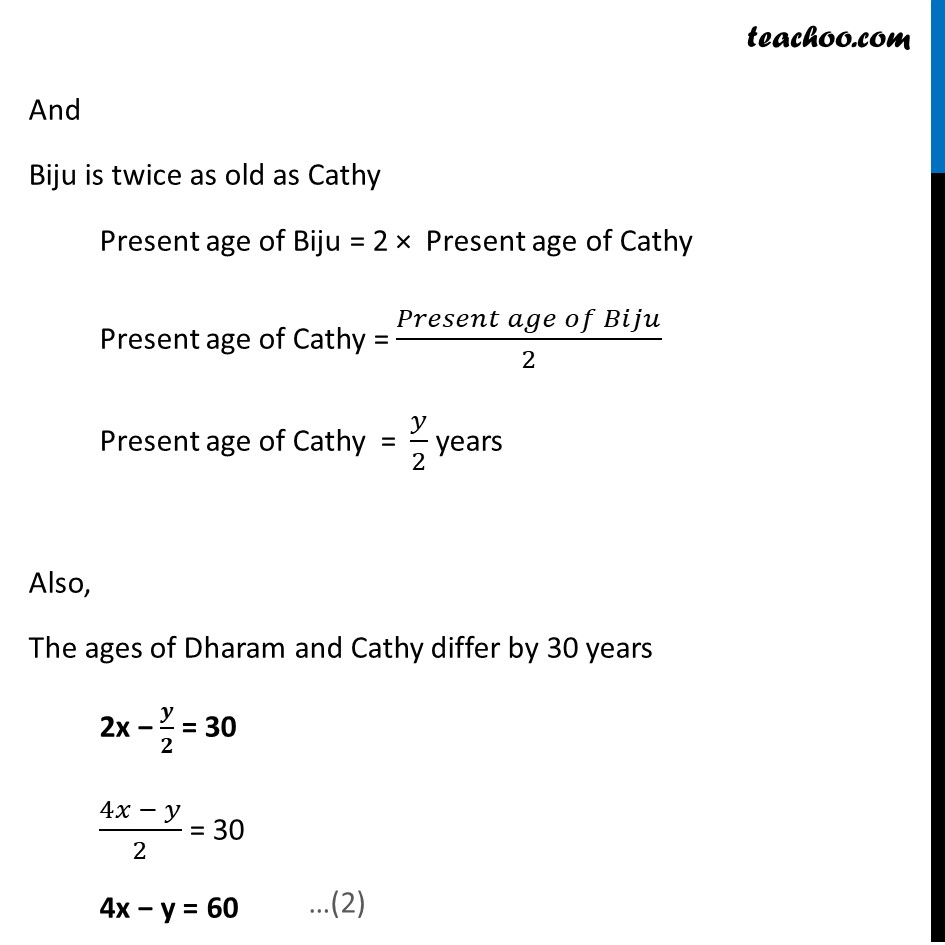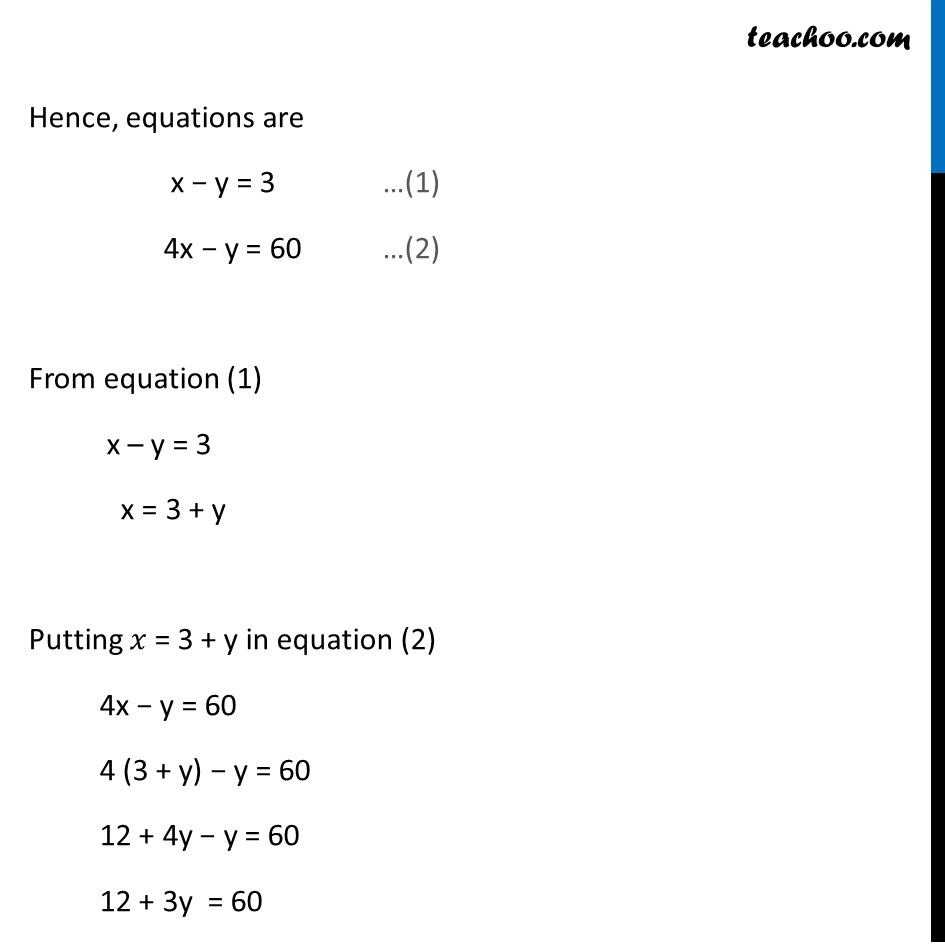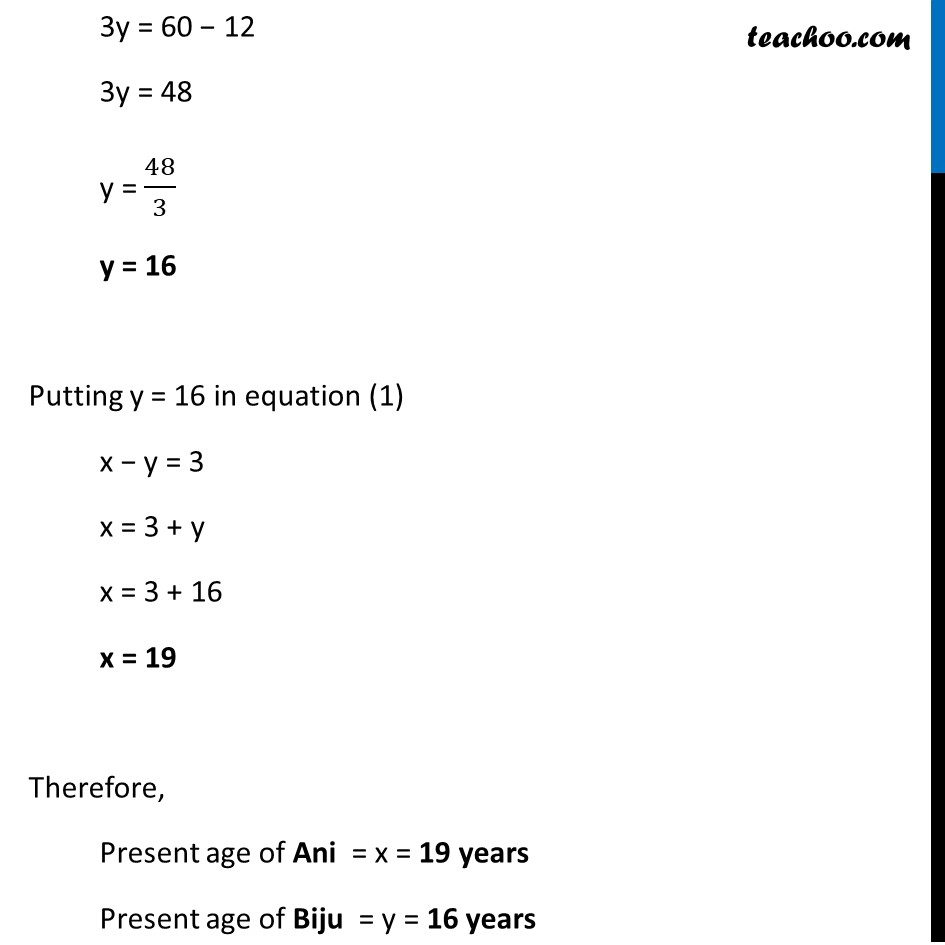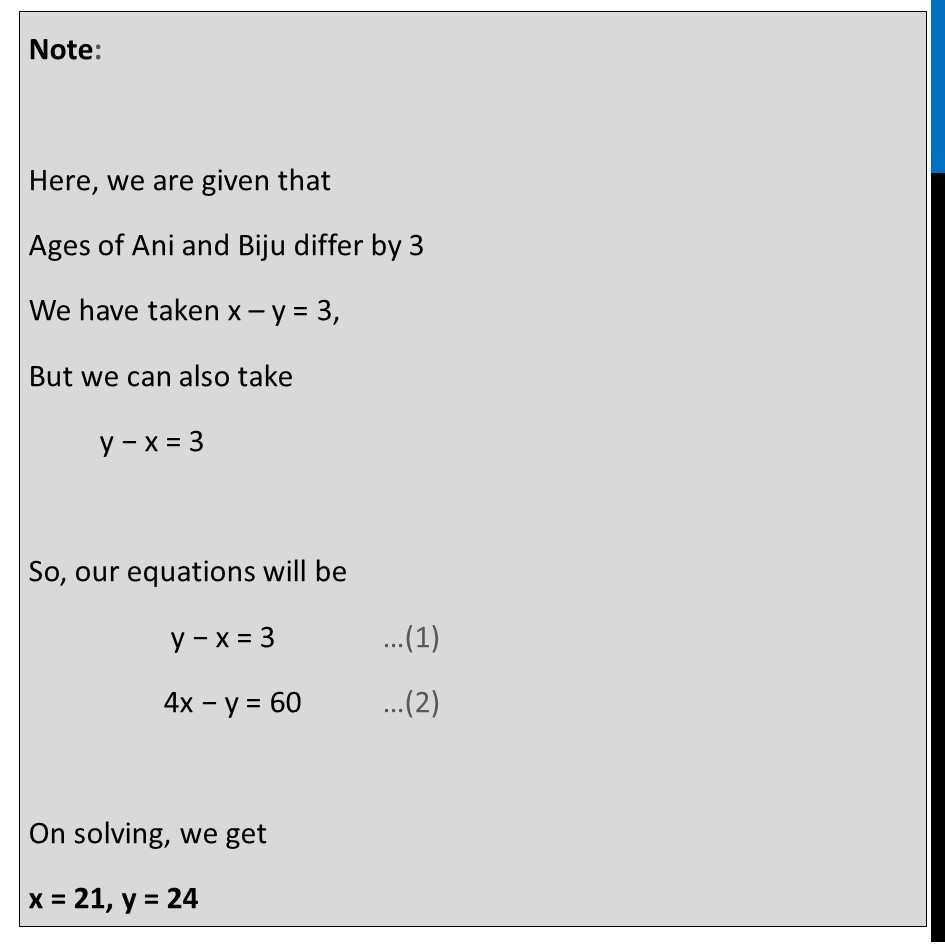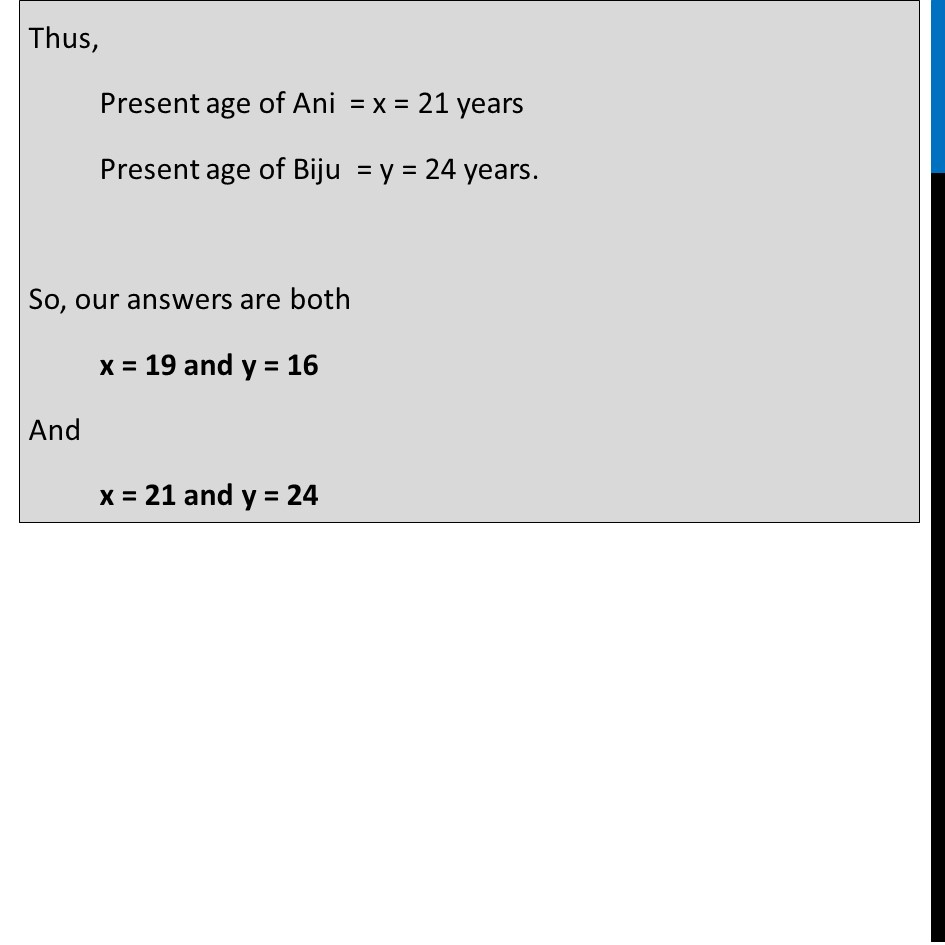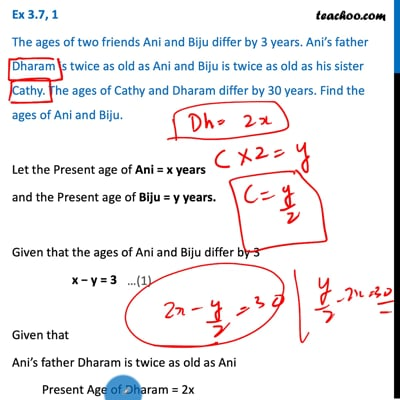This video is only available for Teachoo black users

Maths Crash Course - Live lectures + all videos + Real time Doubt solving!

### Transcript

Ex 3.7, 1 The ages of two friends Ani and Biju differ by 3 years. Ani’s father Dharam is twice as old as Ani and Biju is twice as old as his sister Cathy. The ages of Cathy and Dharam differ by 30 years. Find the ages of Ani and Biju. Let the Present age of Ani = x years and the Present age of Biju = y years. Given that the ages of Ani and Biju differ by 3 x − y = 3 Given that Ani’s father Dharam is twice as old as Ani Present Age of Dharam = 2x And Biju is twice as old as Cathy Present age of Biju = 2 × Present age of Cathy Present age of Cathy = (𝑃𝑟𝑒𝑠𝑒𝑛𝑡 𝑎𝑔𝑒 𝑜𝑓 𝐵𝑖𝑗𝑢)/2 Present age of Cathy = 𝑦/2 years Also, The ages of Dharam and Cathy differ by 30 years 2x − 𝒚/𝟐 = 30 (4𝑥 − 𝑦)/2 = 30 4x − y = 60 Hence, equations are x − y = 3 …(1) 4x − y = 60 …(2) From equation (1) x – y = 3 x = 3 + y Putting 𝑥 = 3 + y in equation (2) 4x − y = 60 4 (3 + y) − y = 60 12 + 4y − y = 60 12 + 3y = 60 3y = 60 − 12 3y = 48 y = 48/3 y = 16 Putting y = 16 in equation (1) x − y = 3 x = 3 + y x = 3 + 16 x = 19 Therefore, Present age of Ani = x = 19 years Present age of Biju = y = 16 years Note: Here, we are given that Ages of Ani and Biju differ by 3 We have taken x – y = 3, But we can also take y − x = 3 So, our equations will be y − x = 3 …(1) 4x − y = 60 …(2) On solving, we get x = 21, y = 24 Thus, Present age of Ani = x = 21 years Present age of Biju = y = 24 years. So, our answers are both x = 19 and y = 16 And x = 21 and y = 24